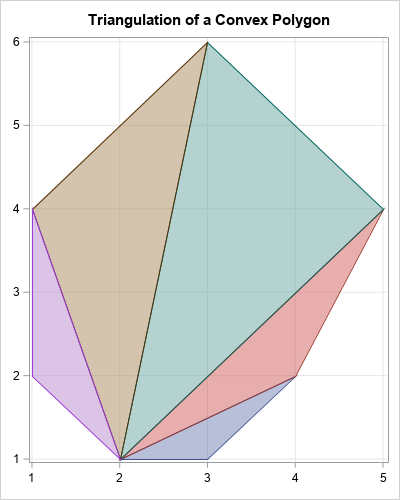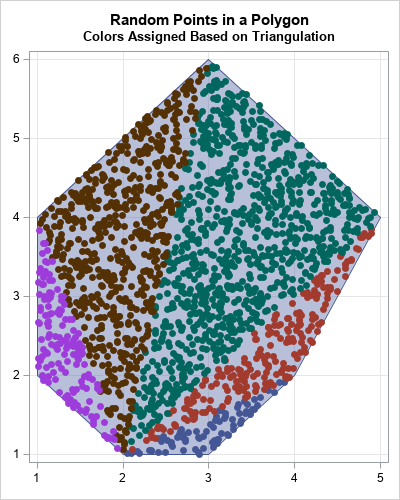The triangulation theorem for polygons says that every simple polygon can be triangulated. In fact, if the polygon has V vertices, you can decompose it into V-2 non-overlapping triangles. In this article, a "polygon" always means a simple polygon. Also, a "random point" means one that is drawn at random from the uniform distribution.

The triangularization of a polygon is useful in many ways, but one application is to generate uniform random points in a polygon or a collection of polygons. Because polygons can be decomposed into triangles, the problem reduces to a simpler one: Given a list of k triangles, generate uniform random points in the union of the triangles. I have already shown how to generate random points in a triangle, so you can apply this method to generate random points in a polygon or collection of polygons.

### An algorithm to generate random points in a polygon

Suppose that a polygon or any other planar region is decomposed into k triangles T1, T2, ..., Tk. If you want to generate N random points uniformly in the region, the number of points in any triangle should be proportional to the area of the triangle divided by the total area of the polygon.

One way to accomplish this is to use a two-step process. First, choose a triangle by using a probability proportional to the relative areas. Next, generate a random point in that triangle. This two-step approach is suitable for the SAS DATA step. At the end of this process, you have generated Ni observations in triangle Ti.

An equivalent formulation is to realize that the vector {N1, N2, ..., Nk} is a random draw from the multinomial distribution with parameters p = {p1, p2, ..., pk}, where pi = Area(Ti) / (Σj Area(Tj)). This second formulation is better for a vector languages such as the SAS/IML language.

Therefore, the following algorithm generates random points in a polygon:

1. Decompose the polygon into triangles T1, T2, ..., Tk.
2. Compute the areas: Ai = Area(Ti).
3. Generate one random draw from the multinomial distribution with probability vector p = {p1, p2, ..., pk}, where pi = Ai / (Σj Aj). This gives a vector of numbers {N1, N2, ..., Nk}.
4. Use the algorithm from the previous article to generate Ni random points in the triangle Ti.

Notice that Steps 2-4 of this algorithm apply to ANY collection of triangles. To make the algorithm flexible, I will implement the first step (the decomposition) in one function and the remaining steps in a second function.

### Triangulate a convex polygon in SAS

There are various general methods for triangulating a polygon, but for convex polygons, there is a simple method. From among the V vertices, choose any vertex and call it P1. Enumerate the remaining vertices consecutively in a counter-clockwise direction: P2, P3, ..., Pk, where k = V-2. Because the polygon is convex, the following triangles decompose the polygon:

• T1 = {P1, P2, P3}
• T2 = {P1, P3, P4}, and so forth, up to
• Tk-2 = {P1, Pk-1, Pk}

The following SAS/IML function decomposes a convex polygon into triangles. The triangles are returned in a SAS/IML list. The function is called on a convex hexagon and the resulting decomposition is shown below. The function uses the PolyIsConvex function, which is part of the Polygon package. You can download and install the Polygon package. You need to load the Polygon package before you call the function.

```/* assume the polygon package is installed */ proc iml; package load polygon; /* load the polygon package */   /* Decompose a convex polygon into triangles. Return a list that contains the vertices for the triangles. This function uses a function in the Polygon package, which must be loaded. */ start TriangulateConvex(P); /* input parameter(N x 2): vertices of polygon */ isConvex = PolyIsConvex(P); if ^isConvex then return ( [] ); /* The polygon is not convex */ numTri = nrow(P) - 2; /* number of triangles in convex polygon */ L = ListCreate(numTri); /* create list to store triangles */ idx = 2:3; do i = 1 to ListLen(L); L\$i = P[1,] // P[idx,]; idx = idx + 1; end; return (L); finish;   /* Specify a convex polygon and visualize the triangulation. */ P = { 2 1 , 3 1 , 4 2 , 5 4 , 3 6 , 1 4 , 1 2 }; L = TriangulateConvex(P);```To illustrate the process, I've included a graph that shows a decomposition of the convex hexagon into triangles. The triangles are returned in a list. The next section shows how to generate uniform points at random inside the union of the triangles in this list.

### Generate random points in a collection of triangles

This section generates random points in a union of triangles. The following function takes two arguments: the number of points to generate (N) and a list of triangles (L). The algorithm computes the relative areas of the triangles and uses them to determine the probability that a point will be generated in each. It then uses the RandUnifTriangle function from the previous article to generate the random points.

```/* Given a list of triangles (L), generate N random points in the union, where the number of points is proportional to Area(triangle) / Area(all triangles)   This function uses functions in the Polygon package, which must be loaded. */ start RandUnifManyTriangles(N, L); numTri = ListLen(L); /* compute areas of each triangle in the list */ AreaTri = j(1, numTri,.); /* create vector to store areas */ do i = 1 to numTri; AreaTri[i] = PolyArea(L\$i); /* PolyArea is in the Polygon package */ end; /* Numbers of points in the triangles are multinomial with probability proportional to Area(triangle)/Area(polygon) */ NTri = RandMultinomial(1, N, AreaTri/sum(AreaTri)); cumulN = 0 || cusum(NTri); /* cumulative counts; use as indices */ z = j(N, 3, .); /* columns are (x,y,TriangleID) */ do i = 1 to numTri; k = (cumulN[i]+1):cumulN[i+1]; /* the next NTri[i] elements */ z[k, 1:2] = RandUnifTriangle(L\$i, NTri[i]); z[k, 3] = i; /* store the triangle ID */ end; return z; finish;   /* The RandUnifTriangle function is defined at https://blogs.sas.com/content/iml/2020/10/19/random-points-in-triangle.html */ load module=(RandUnifTriangle); call randseed(12345); N = 2000; z = RandUnifManyTriangles(N, L);```

The z vector is an N x 3 matrix. The first two columns contain the (x,y) coordinates of N random points. The third column contains the ID number (values 1,2,...,k) that indicates the triangle that each point is inside of. You can use the PolyDraw function in the Polygon package to visualize the distribution of the points within the polygon:

```title "Random Points in a Polygon"; title2 "Colors Assigned Based on Triangulation"; call PolyDraw(P, z);```The color of each point indicates which triangle the point is inside. You can see that triangles with relatively small areas (blue and purple) have fewer points than triangles with larger areas (green and brown).

### Summary

In summary, this article shows how to generate random points inside a planar polygon. The first step is to decompose the polygon into triangles. You can use the relative areas of the triangles to determine the probability that a random point is in each triangle. Finally, you can generate random points in the union of the triangles. (Note: The algorithm works for any collection of planar triangles.)

This article uses functions in the Polygon package. Installing and loading a package is a way to define a set of related functions that you want to share. It is an alternative to using %INCLUDE to include the module definitions into your program.

Share